# Part I - Shortest Path

## Shortest path

Is a problem of finding a path between two nodes, such that the sum of the weights of its constituent edges is minimized.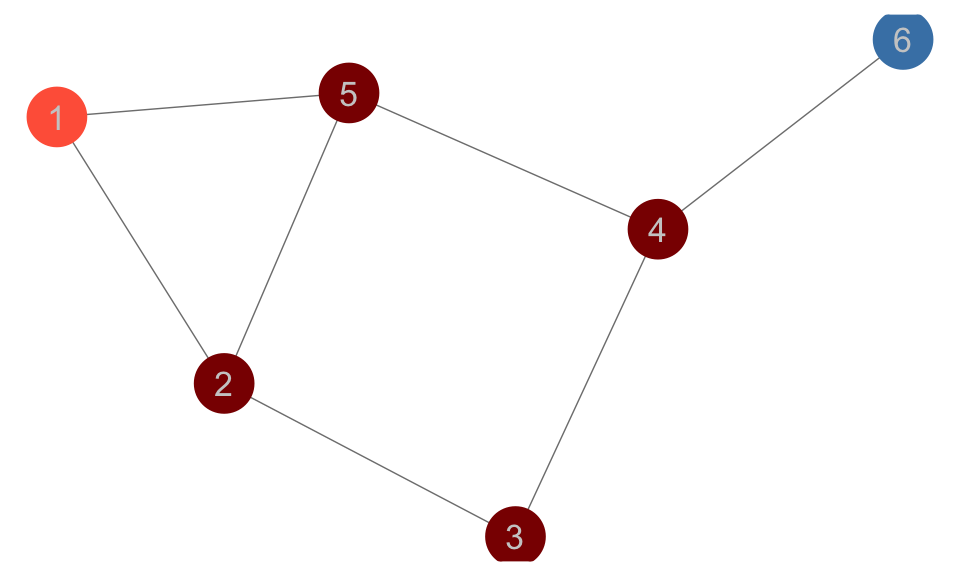• Paths between 1 and 6: 1-5-4-6 and 1-2-3-4-6.
• Which is the shortest?

## Shortest path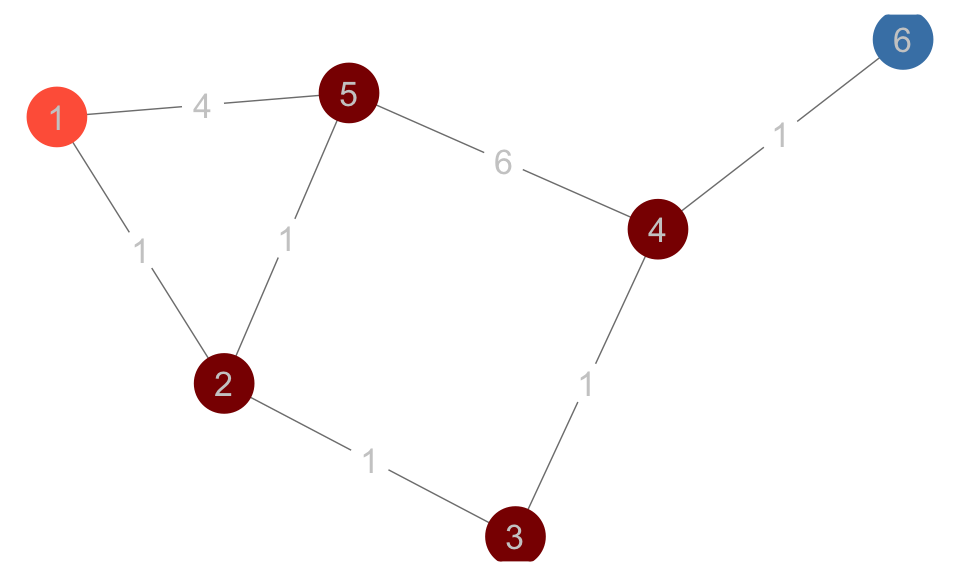• What is the shortest path now?
• How can we programmatically calculate the shortest path between any 2 nodes for any graph?

## Dijkstra - Initialization

\begin{aligned} &InitializeSingleSource(G,s)\\ &\qquad for \space each \space vertex \space v \in G.V \\ &\qquad \qquad v.d = \infty \\ &\qquad \qquad v.\pi = NIL \\ &\qquad s.d = 0 \\ \end{aligned}

## Dijkstra - Relax function

\begin{aligned} &Relax(u,v,w)\\ &\qquad if \space v.d > u.d + w(u,v)\\ &\qquad \qquad v.d = u.d + w(u,v)\\ &\qquad \qquad v.\pi = u \\ \end{aligned}

## Dijkstra - Main algorithm

\begin{aligned} &Dijkstra(G,w,s)\\ &\qquad InitializeSingleSource(G,s)\\ &\qquad S = \emptyset\\ &\qquad Q = G.V \\ &\qquad while \space Q \neq \emptyset\\ &\qquad \qquad u = ExtractMin(Q) \\ &\qquad \qquad S = S \cup \{u\} \\ &\qquad \qquad for \space each \space v \in G.Adj[u]\\ &\qquad \qquad \qquad Relax(u,v,w)\\ \end{aligned}

## Dijkstra Visualization Step 1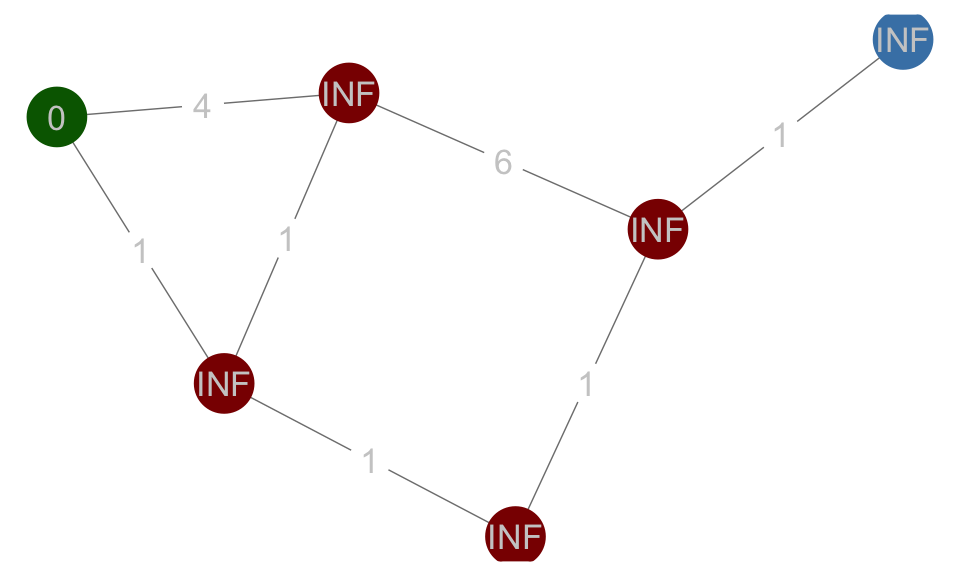Green - current node, Red - unvisited node, Blue - target node. Assign distance to the start node as 0, and INF to all others. Assign the start node as the current node.

## Dijkstra Visualization Step 2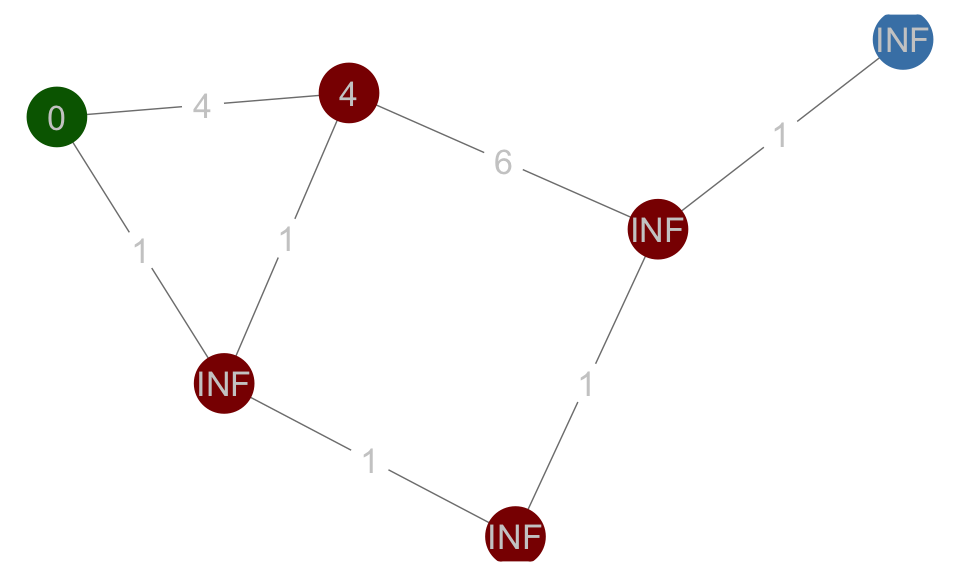Updating the distance to neighbors of the current node.

## Dijkstra Visualization Step 3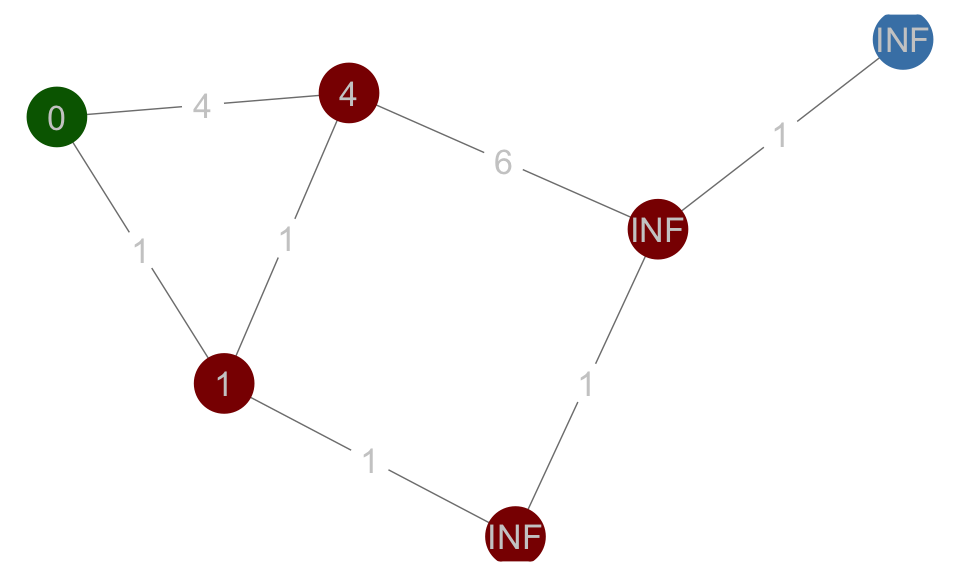Updating the distance to neighbors of the current node.

## Dijkstra Visualization Step 4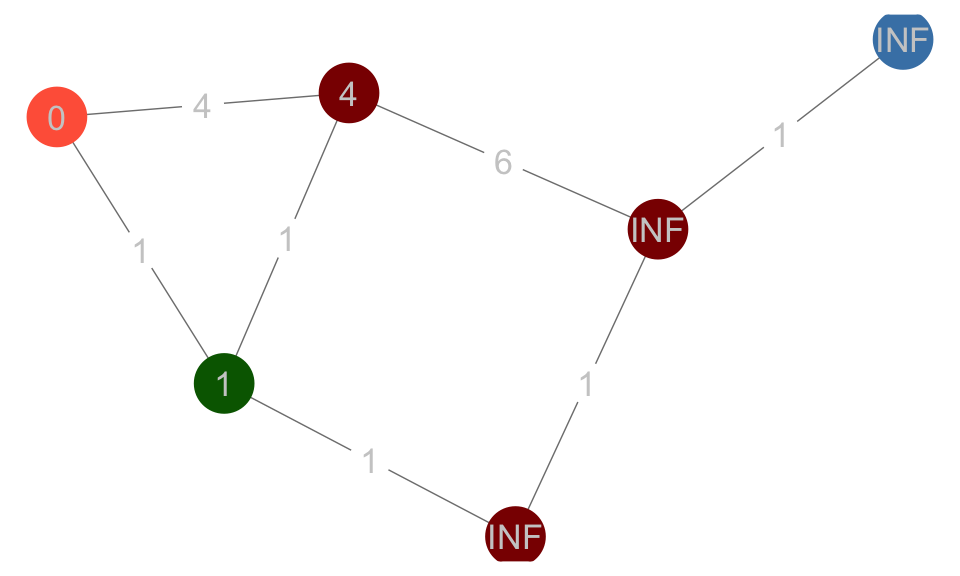Make the second node as current node. Mark the first node is visited.